International
Tables for
Crystallography
Volume B
Reciprocal space
Edited by U. Shmueli

International Tables for Crystallography (2010). Vol. B, ch. 2.5, pp. 344-348   | 1 | 2 |

## Section 2.5.3.4.1. General remarks

M. Tanakaf

#### 2.5.3.4.1. General remarks

| top | pdf |

Incommensurately modulated crystals do not have three-dimensional lattice periodicity. The crystals, however, recover lattice periodicity in a space higher than three dimensions. de Wolff (1974, 1977) showed that one-dimensional displacive and substitutionally modulated crystals can be described as a three-dimensional section of a (3 + 1)-dimensional periodic crystal. Janner & Janssen (1980a,b) developed a more general approach for describing a modulated crystal with n modulations as (3 + n)-dimensional periodic crystals (n = 1, 2, …). Yamamoto (1982) derived a general structure-factor formula for n-dimensionally modulated crystals (n = 1, 2, …), which holds for both displacive and substitutionally modulated crystals. Tables of the (3 + 1)-dimensional space groups for one-dimensional incommensurately modulated crystals were given by de Wolff et al. (1981), where the wavevector of the modulation was assumed to lie in the c direction. Later, some corrections to the tables were made by Yamamoto et al. (1985). The analysis of incommensurately modulated crystals using (3 + 1)-dimensional space groups has become familiar in the field of X-ray structure analysis.

Fung et al. (1980) applied the CBED method to the study of incommensurately modulated transition-metal dichalcogenides. Steeds et al. (1985) applied the LACBED method (Tanaka et al., 1980) to the study of incommensurately modulated crystals of NiGe1−xPx. Tanaka et al. (1988, pp. 74–81) examined the symmetries of the incommensurate and fundamental reflections appearing in the CBED patterns obtained from the incommensurately modulated crystals of Sr2Nb2O7 and Mo8O23. Terauchi & Tanaka (1993) clarified theoretically the interrelation between the symmetries of CBED patterns and the (3 + 1)-dimensional point-group symbols for incommensurately modulated crystals and verified experimentally the theoretical results for Sr2Nb2O7 and Mo8O23. Terauchi et al. (1994) investigated dynamical extinction for the (3 + 1)-dimensional space groups. They clarified that approximate dynamical extinction lines appear in CBED discs of the reflections caused by incommensurate modulations when the amplitudes of the incommensurate modulation waves are small. They tabulated the dynamical extinction lines appearing in the CBED discs for all the (3 + 1)-dimensional space groups of the incommensurately modulated crystals. The tables were stored in the British Library Document Supply Centre as Supplementary Publication No. SUP 71810 (65 pp.). They showed an example of the dynamical extinction lines obtained from Sr2Nb2O7. The point- and space-group determinations of the (3 + 1)-dimensional crystals are described compactly in the book by Tanaka et al. (1994, pp. 156–205).

Fig. 2.5.3.17illustrates (3 + 1)-dimensional descriptions of a crystal structure without modulation (a), a one-dimensional displacive modulated structure (b) and a one-dimensional substitutionally modulated structure (c). The arrows labelled a1a3 (a, b and c) and a4 indicate the (3 + 1)-dimensional crystal axes. The horizontal line labelled R3 represents the three-dimensional space (external space). In the (3 + 1)-dimensional description, an atom is not located at a point as in the three-dimensional space, but extends as a string along the fourth direction a4 perpendicular to the three-dimensional space R3. The shaded parallelogram is a unit cell in the (3 + 1)-dimensional space. The unit cell contains two atom strings in this case. In the case of no modulations, the atoms are shown as straight strings, as shown in Fig. 2.5.3.17(a). For a displacive modulation, atoms are expressed by wavy strings periodic along the fourth direction a4 as shown in Fig. 2.5.3.17(b). The width of the atom strings indicates the spread of the atoms in R3. The atom positions of the modulated structure in R3 are given as a three-dimensional (R3) section of the atom strings in the (3 + 1)-dimensional space. A substitutional modulation, which is described by a modulation of the atom form factor, is expressed by atom strings with a density modulation along the direction a4 as shown in Fig. 2.5.3.17(c).Figure 2.5.3.17 | top | pdf |The (3 + 1)-dimensional description of one-dimensionally modulated crystals. Atoms are shown as strings along the fourth direction a4. (a) No modulation, shown as straight strings. (b) Displacive modulation, shown as wavy strings. (c) Amplitude modulation with varying-density strings.

The diffraction vector G is written as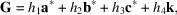where a set of h1h2h3h4 is a (3 + 1)-dimensional reflection index, and a*, b* and c* are the reciprocal-lattice vectors of the real-lattice vectors a, b and c of the average structure. The modulation vector k is written as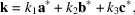where one coefficient ki (i = 1–3) is an irrational number and the others are rational. Fig. 2.5.3.18(a) shows a diffraction pattern of a crystal with an incommensurate modulation wavevector k1a* (k2 and k3 = 0). Large and small black spots show the fundamental reflections and incommensurate reflections, respectively, only the first-order incommensurate reflections being shown. It should be noted that the diffraction pattern of a modulated crystal is obtained by a projection of the Fourier transform of the (3 + 1)-dimensional periodic structure. Fig. 2.5.3.18(b) is assumed to be the Fourier transform of Fig. 2.5.3.17(b). Incommensurate reflections are obtained by a projection of the reciprocal-lattice points onto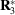.Figure 2.5.3.18 | top | pdf |(a) Schematic diffraction pattern from a modulated crystal. As an example, the wave number vector of modulation is assumed to be k1a*, k1 being an irrational number. Large and small spots denote fundamental and incommensurate reflections, respectively. (b) Incommensurate reflections are obtained by a projection of the Fourier transform of a (3 + 1)-dimensional periodic structure.

The displacive modulation is expressed by the atom displacement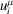with x4. The structure factor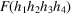for the (3 + 1)-dimensional crystal with a displacive modulation is given by de Wolff (1974, 1977) as follows: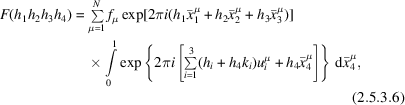where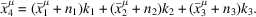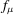and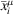(i = 1–3) are, respectively, the atom form factor and the ith component of the position of the μth atom in the unit cell of the average structure. The symbolis the ith component of the displacement of the μth atom. Since the atom in the (3 + 1)-dimensional space is continuous along a4 and discrete along R3, the structure factor is expressed by summation in R3 and integration along a4 as seen in equation (2.5.3.6). The integration implies that the sum for the atoms with displacements is taken over the infinite number of unit cells of the average structure. That is, equation (2.5.3.6) is the structure factor for a unit cell with the lattice parameter of an infinite length in R3 along the direction of the modulation wavevector k.

CBED patterns are obtained from a finite area of a specimen crystal. For the symmetry analysis of CBED patterns obtained from modulated structures, the effect of the finite size was considered by Terauchi & Tanaka (1993). The integration over a unit-cell length along a4 in equation (2.5.3.6) is rewritten in the following way with the summation over a finite number of three-dimensional sections of the atom strings: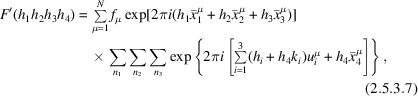where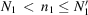,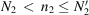and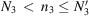,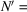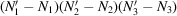being the number of unit cells of the average structure included in a specimen volume from which CBED patterns are taken.

The substitutional modulation arises from a periodic variation of the site-occupation probability of the atoms. This modulation is expressed by a modulation of the atom form factorwith x4. The structure factor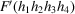for a finite-size crystal is written as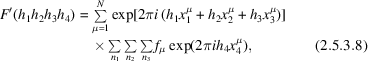where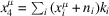.

### References

Fung, K. K., Steeds, J. W. & Eades, J. A. (1980). Application of convergent beam electron diffraction to study the stacking of layers in transition-metal dichalcogenides. Physica (Utrecht), B99, 47–50.
Janner, A. & Janssen, T. (1980a). Symmetry of incommensurate crystal phases. I. Commensurate basic structures. Acta Cryst. A36, 399–408.
Janner, A. & Janssen, T. (1980b). Symmetry of incommensurate crystal phases. II. Incommensurate basic structure. Acta Cryst. A36, 408–415.
Steeds, J. W., Bird, D. M., Eaglesham, D. J., Mckernen, S., Vincent, R. & Withers, R. L. (1985). Study of modulated structures by transmission electron microscopy. Ultramicroscopy, 18, 97–110.
Tanaka, M., Saito, P., Ueno, K. & Harada, Y. (1980). Large angle convergent-beam electron diffraction. J. Electron. Microsc. 29, 408–412.
Tanaka, M., Terauchi, M. & Kaneyama, T. (1988). Convergent-Beam Electron Diffraction II. Tokyo: JEOL Ltd.
Tanaka, M., Terauchi, M. & Tsuda, K. (1994). Convergent-Beam Electron Diffraction III. Tokyo: JEOL–Maruzen.
Terauchi, M., Takahashi, M. & Tanaka, M. (1994). Convergent-beam electron diffraction study of incommensurately modulated crystals. II. (3+1)-dimensional space groups. Acta Cryst. A50, 566–574.
Terauchi, M. & Tanaka, M. (1993). Convergent-beam electron diffraction study of incommensurately modulated crystals. I. (3+1)-dimensional point groups. Acta Cryst. A49, 722–729.
Wolff, P. M. de (1974). The pseudo-symmetry of modulated crystal structures. Acta Cryst. A30, 777–785.
Wolff, P. M. de (1977). Symmetry operations for displacively modulated structures. Acta Cryst. A33, 493–497.
Wolff, P. M. de, Janssen, T. & Janner, A. (1981). The superspace groups for incommensurate crystal structures with a one-dimensional modulation. Acta Cryst. A37, 625–636.
Yamamoto, A. (1982). Structure factor of modulated crystal structures. Acta Cryst. A38, 87–92.
Yamamoto, A., Janssen, T., Janner, A. & de Wolff, P. M. (1985). A note on the superspace groups for one-dimensionally modulated structures. Acta Cryst. A41, 528–530.